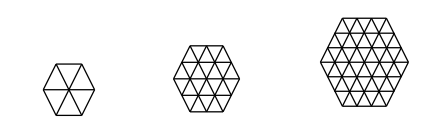# Partitioning A Hexagon

Probability Level 2Following the pattern of the first 3 figures above, how many little triangles are there in the $9^\text{th}$ figure?

×